Previous Page | Next Page

 Language Reference

# Overview

This chapter describes all operators, statements, functions, and subroutines that can be used in SAS/IML software. All necessary details, such as arguments and operands, are included.

This chapter is divided into two sections. The first section contains operator descriptions. They are in alphabetic order according to the name of the operator. The second section contains descriptions of statements, functions, and subroutines also arranged alphabetically by name.

The following tables list all statements, functions, and subroutines available in SAS/IML software grouped by functionality.

### Scalar Functions

 ABS Function takes the absolute value EXP Function calculates the exponential INT Function truncates a value LOG Function takes the natural logarithm MOD Function computes the modulo (remainder) NORMAL Function generates a pseudo-random normal deviate SQRT Function calculates the square root UNIFORM Function generates pseudo-random uniform deviates

### Reduction Functions

 MAX Function finds the maximum value of a matrix MIN Function finds the smallest element of a matrix SSQ Function calculates the sum of squares of all elements SUM Function sums all elements

### Matrix Inquiry Functions

 ALL Function checks for all nonzero elements ANY Function checks for any nonzero elements CHOOSE Function conditionally chooses and changes elements LOC Function finds nonzero elements of a matrix NCOL Function finds the number of columns of a matrix NLENG Function finds the size of an element NROW Function finds the number of rows of a matrix TYPE Function determines the type of a matrix

### Matrix Sorting and BY-Group Processing Calls

 SORT Call sorts a matrix by specified columns SORTNDX Call creates a sorted index for a matrix UNIQUEBY Function finds locations of unique BY groups in a sorted or indexed matrix

### Matrix Reshaping Functions

 BLOCK Function forms block-diagonal matrices BTRAN Function computes block transpose DIAG Function creates a diagonal matrix DO Function produces an arithmetic series I Function creates an identity matrix INSERT Function inserts one matrix inside another J Function creates a matrix of identical values REMOVE Function discards elements from a matrix REPEAT Function creates a new matrix of repeated values SHAPE Function reshapes and repeats values SQRSYM Function converts a symmetric matrix to a square matrix SYMSQR Function converts a square matrix to a symmetric matrix T Function transposes a matrix VECDIAG Function creates a vector from a diagonal

### Character Functionality

 BYTE Function translates numbers to ordinal characters CHANGE Call replaces text CHAR Function produces a character representation of a matrix CONCAT Function Concatenates elementwise strings CSHAPE Function reshapes and repeats character values LENGTH Call finds the lengths of character matrix elements NAME Function lists the names of arguments NUM Function produces a numeric representation of a character matrix ROWCAT Function concatenates rows without using blank compression ROWCATC Function concatenates rows by using blank compression SUBSTR Function takes substrings of matrix elements

### Random Number Generation Functionality

 RANDGEN Call generates random numbers from specified distributions RANDSEED Call initializes seed for subsequent RANGEN calls

### Statistical Functionality

 BRANKS Function computes bivariate ranks CUSUM Function calculates cumulative sums DESIGN Function creates a design matrix DESIGNF Function creates a full-rank design matrix GEOMEAN Function calculates geometric means HADAMARD Function creates a Hadamard matrix HARMEAN Function calculates harmonic means IPF Call performs an iterative proportional fit of a contingency table LAV Call performs linear least absolute value regression by solving the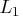norm minimization problem LMS Call performs robust regression LTS Call performs robust regression MAD Function finds the univariate (scaled) median absolute deviation MARG Call evaluates marginal totals in a multiway contingency table MAXQFORM Call computes the subsets of a matrix system that maximize the quadratic form MCD Call finds the minimum covariance determinant estimator MVE Call finds the minimum volume ellipsoid estimator OPSCAL Function rescales qualitative data to be a least squares fit to qualitative data RANK Function ranks elements of a matrix RANKTIE Function ranks matrix elements by using tie-averaging SEQSCALE Call perform discrete sequential tests SEQSHIFT Call perform discrete sequential tests SEQTESTS Calls perform discrete sequential tests SWEEP Function sweeps a matrix

### Time Series Functionality

 ARMACOV Call computes an autocovariance sequence for an ARMA model ARMALIK Call computes the log likelihood and residuals for an ARMA model ARMASIM Function simulates an ARMA series CONVEXIT Function calculates convexity of a noncontingent cash flow COVLAG Function computes autocovariance estimates for a vector time series DURATION Function calculates modified duration of a noncontingent cash flow FARMACOV Call computes the autocovariance function for an ARFIMA(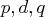) process FARMAFIT Call estimate the parameters of an ARFIMA() model FARMALIK Call computes the log-likelihood function of an ARFIMA() model FARMASIM Call generates an ARFIMA() process FDIF Call obtain a fractionally differenced process FORWARD Function calculates forward rates KALCVF Call computes the one-step prediction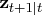and the filtered estimate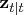, as well as their covariance matrices. The call uses forward recursions, and you can also use it to obtain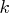-step estimates. KALCVS Call uses backward recursions to compute the smoothed estimate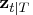and its covariance matrix,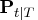, where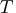is the number of observations in the complete data set. KALDFF Call computes the one-step forecast of state vectors in an SSM by using the diffuse Kalman filter. The call estimates the conditional expectation of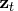, and it also estimates the initial random vector,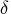, and its covariance matrix. KALDFS Call computes the smoothed state vector and its mean square error matrix from the one-step forecast and mean square error matrix computed by KALDFF. PV Function calculates present value RATES Function converts interest rates from one base to another SPOT Function calculates spot rates TSBAYSEA Call performs Bayesian seasonal adjustment modeling TSDECOMP Call analyzes nonstationary time series by using smoothness priors modeling TSMLOCAR Call analyzes nonstationary or locally stationary time series by using the minimum AIC procedure TSMLOMAR Call analyzes nonstationary or locally stationary multivariate time series by using the minimum AIC procedure TSMULMAR Call estimates VAR processes by using the minimum AIC procedure TSPEARS Call analyzes periodic AR models with the minimum AIC procedure TSPRED Call provides predicted values of univariate and multivariate ARMA processes when the ARMA coefficients are input TSROOT Call calculates AR and MA coefficients from the characteristic roots of the model or calculates the characteristic roots of the model from the AR and MA coefficients TSTVCAR Call analyzes time series that are nonstationary in the covariance function TSUNIMAR Call determines the order of an AR process with the minimum AIC procedure and estimates the AR coefficients VARMACOV Call computes the theoretical cross-covariance matrices for a stationary VARMA(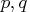) model VARMALIK Call computes the log-likelihood function for a VARMA() model VARMASIM Call generates VARMA(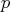,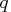) time series VNORMAL Call generates multivariate normal random series VTSROOT Call computes the characteristic roots for a VARMA() model YIELD Function calculates yield-to-maturity of a cash-flow stream

### Numerical Analysis Functionality

 BSPLINE Function computes B-spline basis FFT Function performs the finite Fourier transform IFFT Function computes the inverse finite Fourier transform JROOT Function computes the first nonzero roots of a Bessel function of the first kind and the derivative of the Bessel function at each root ODE Call performs numerical integration of vector differential equations of the form ORPOL Function generates orthogonal polynomials on a discrete set of points ORTVEC Call provides columnwise orthogonalization by the Gram-Schmidt process and stepwise QR decomposition by the Gram-Schmidt process POLYROOT Function finds zeros of a real polynomial PRODUCT Function multiplies matrices of polynomials QUAD Call performs numerical integration of scalar functions in one dimension over infinite, connected semi-infinite, and connected finite intervals RATIO Function divides matrix polynomials SPLINE Call fits a cubic spline to points SPLINEC Call fits a cubic spline to points SPLINEV Function evaluates a cubic spline at new data points TPSPLINE Call computes thin-plate smoothing splines TPSPLNEV Call evaluates the thin-plate smoothing spline at new data points

### Linear Algebra Functionality

 APPCORT CALL completes orthogonal decomposition COMPORT Call completes orthogonal decomposition by Householder transformations CVEXHULL Function finds a convex hull of a set of planar points DET Function computes the determinant of a square matrix ECHELON Function reduces a matrix to row-echelon normal form EIGEN Call computes eigenvalues and eigenvectors EIGVAL Function computes eigenvalues EIGVEC Function computes eigenvectors GENEIG Call computes eigenvalues and eigenvectors of a generalized eigenproblem GINV Function computes the generalized inverse GSORTH Call computes the Gram-Schmidt orthonormalization HALF Function computes Cholesky decomposition HANKEL Function generates a Hankel matrix HDIR Function performs a horizontal direct product HERMITE Function reduces a matrix to Hermite normal form HOMOGEN Function solves homogeneous linear systems INV Function produces the inverse INVUPDT Function updates a matrix inverse ITSOLVER Call solves a sparse general linear system by iteration LUPDT Call provides updating and downdating for rank deficient linear least squares solutions, complete orthogonal factorization, and Moore-Penrose inverses QR Call produces the QR decomposition of a matrix by Householder transformations RDODT Call downdates and updates QR and Cholesky decompositions ROOT Function performs the Cholesky decomposition of a matrix RUPDT Call updates QR and Cholesky decompositions RZLIND Call updates QR and Cholesky decompositions SOLVE Function solves a system of linear equations SOLVELIN Call solves a sparse symmetric system of linear equations by direct decomposition SVD Call computes the singular value decomposition TEIGEN Call computes the eigenvalues and eigenvectors of square matrices TEIGVAL Function computes eigenvalues of square matrices TEIGVEC Function computes eigenvectors of square matrices TOEPLITZ Function generates a Toeplitz or block-Toeplitz matrix TRACE Function sums diagonal elements TRISOLV Function solves linear systems with triangular matrices XMULT Function performs accurate matrix multiplication

### Optimization Subroutines

 LCP Call solves the linear complementarity problem LP Call solves the linear programming problem NLPCG Call performs nonlinear optimization by conjugate gradient method NLPDD Call performs nonlinear optimization by double-dogleg method NLPFDD Call approximates derivatives by finite-differences method NLPFEA Call computes feasible points subject to constraints NLPHQN Call calculates hybrid quasi-Newton least squares NLPLM Call calculates Levenberg-Marquardt least squares NLPNMS Call performs nonlinear optimization by Nelder-Mead simplex method NLPNRA Call performs nonlinear optimization by Newton-Raphson method NLPNRR Call performs nonlinear optimization by Newton-Raphson ridge method NLPQN Call performs nonlinear optimization by quasi-Newton method NLPQUA Call performs nonlinear optimization by quadratic method NLPTR Call performs nonlinear optimization by trust-region method Nonlinear Optimization and Related Subroutines lists of all nonlinear optimization and related subroutines in IML

### Set Functions

 SETDIF Function compares elements of two matrices UNION Function performs unions of sets UNIQUE Function sorts and removes duplicates XSECT Function intersects sets

### Control Statements

 ABORT Statement ends IML APPLY Function applies an IML module CALL Statement calls a subroutine or function DO and END Statements groups statements as a unit DO, Iterative Statement iteratively executes a DO group DO and UNTIL Statement and Clause conditionally executes statements iteratively DO and WHILE Statement and Clause conditionally executes statements iteratively END Statement ends a DO loop or DO statement EXECUTE Call executes SAS statements immediately FINISH Statement denotes the end of a module FORCE Statement (see the description of the SAVE statement) FREE Statement frees matrix storage space GOTO Statement jumps to a new statement IF-THEN/ELSE Statement conditionally executes statement LINK Statement jumps to another statement MATTRIB Statement associates printing attributes with matrices PAUSE Statement interrupts module execution PRINT Statement prints matrix values PURGE Statement removes observations marked for deletion and renumbers records PUSH Call pushes SAS statements into the command input stream QUEUE Call queues SAS statements into the command input stream QUIT Statement exits from IML REMOVE Statement removes matrices from storage RESET Statement sets processing options RESUME Statement resumes execution RETURN Statement returns to caller RUN Statement executes statements in a module SHOW Statement prints system information SOUND Call produces a tone START/FINISH Statements define a module STOP Statement stops execution of statements STORAGE Function lists names of matrices and modules in storage STORE Statement stores matrices and modules in library storage VALSET Call performs indirect assignment VALUE Function assigns values by indirect reference

### Datas Set and File Functionality

 APPEND Statement adds observations to SAS data set CLOSE Statement closes a SAS data set CLOSEFILE Statement closes a file CONTENTS Function returns the variables in a SAS data set CREATE Statement creates a new SAS data set DATASETS Function obtains the names of SAS data sets DELETE Call deletes a SAS data set DELETE Statement marks observations for deletion DO DATA Statement repeats a loop until an end of file occurs EDIT Statement opens a SAS data set for editing FILE Statement opens or points to an external file FIND Statement finds observations INDEX Statement indexes a variable in a SAS data set INFILE Statement opens a file for input INPUT Statement inputs data LIST Statement displays observations of a data set LOAD Statement loads modules and matrices from library storage PUT Statement writes data to an external file READ Statement reads observations from a data set RENAME Call renames a SAS data set REPLACE Statement replaces values in observations and updates observations SAVE Statement saves data SETIN Statement makes a data set current for input SETOUT Statement makes a data set current for output SORT Statement sorts a SAS data set SUMMARY Statement computes summary statistics for SAS data sets USE Statement opens a SAS data set for reading

### Graphics and Window Functions

 DISPLAY Statement displays fields in a display window GBLKVP Call defines a blanking viewport GBLKVPD Call deletes the blanking viewport GCLOSE Call closes the graphics segment GDELETE Call deletes a graphics segment GDRAW Call draws a polyline GDRAWL Call draws individual lines GGRID Call draws a grid GINCLUDE Call includes a graphics segment GOPEN Call opens a graphics segment GPIE Call draws pie slices GPIEXY Call converts from polar to world coordinates GPOINT Call plots points GPOLY Call draws and fills a polygon GPORT Call defines a viewport GPORTPOP Call pops the viewport GPORTSTK Call stacks the viewport GSCALE Call calculates round numbers for labeling axes GSCRIPT Call writes multiple text strings with special fonts GSET Call sets attributes for a graphics segment GSHOW Call shows a graph GSTART Call initializes the graphics system GSTOP Call deactivates the graphics system GSTRLEN Call finds the string length GTEXT and GVTEXT Calls places text horizontally or vertically on a graph GWINDOW Call defines the data window GXAXIS and GYAXIS Calls draws a horizontal or vertical axis PGRAF Call produces scatter plots ODSGRAPH Call renders a graph by using ODS Statistical Graphics WINDOW Statement opens a display window

### Wavelet Analysis Calls

 WAVFT Call computes a specified wavelet transform of one dimensional data WAVGET Call returns requested information encapsulated in a wavelet transform WAVIFT Call inverts a wavelet transform after applying specified thresholding to the detail coefficients WAVPRINT Call displays requested information encapsulated in a wavelet transform WAVTHRSH Call applies specified thresholding to the detail coefficients of a wavelet transform

### Genetic Algorithm Functionality

 GAEND Call terminates genetic algorithm and frees memory resources GAGETMEM Call gets requested members and objective values from current solution population GAGETVAL Call gets objective function values for requested member of current solution population GAINIT Call initializes the initial solution population GAREEVAL Call reevaluates the objective function for all solutions in current population GASETCRO Call specifies a current crossover operator GASETMUT Call specifies a current mutation operator GASETOBJ Call specifies a current objective function GASETSEL Call specifies a current selection parameters GASETUP Function sets up a specific genetic algorithm optimization problem

### Calling External Modules

 MODULEI Call calls an external routine without any return code MODULEIC Call calls an external routine that returns a character MODULEIC Call calls an external routine that returns a numeric valuePrevious Page | Next Page | Top of Page